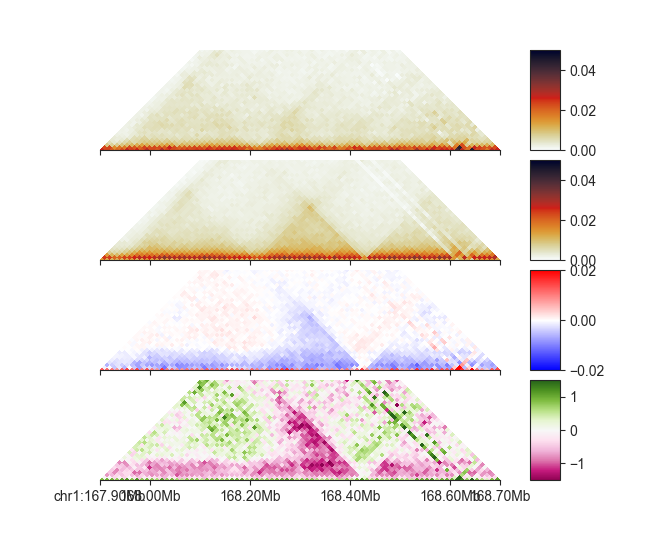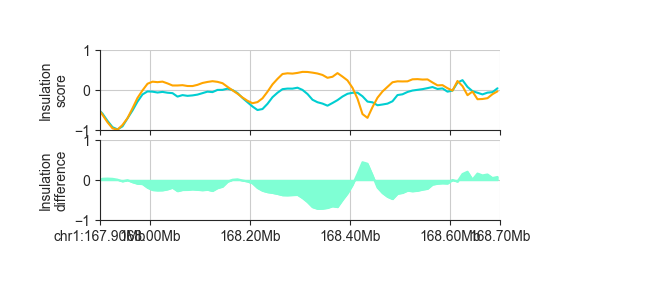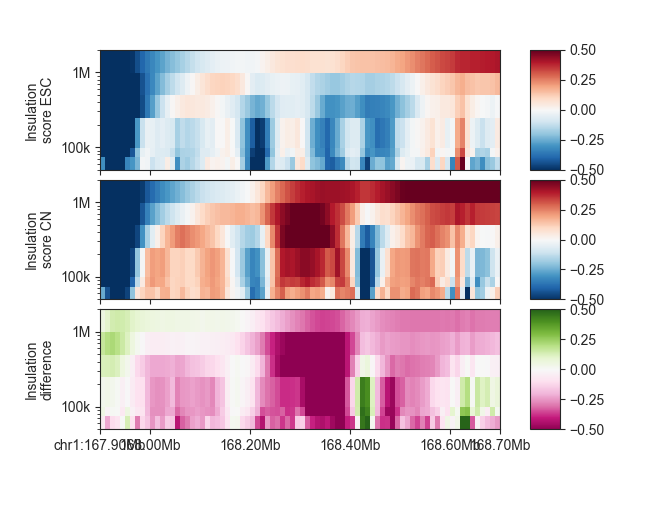# Matrix and score comparisons¶

To follow this tutorial, download the FAN-C example data, for example through our Keeper library, and set up your Python session like this:

```import fanc
import fanc.plotting as fancplot

import logging
logging.basicConfig(level=logging.INFO, format="%(asctime)s %(levelname)s %(message)s")

ins_esc_100kb = ins_esc.score_regions(100000)
ins_cn_100kb = ins_cn.score_regions(100000)
```

Also have a look at the command line documentation at Matrix and score comparisons for the command-line approach to comparisons in FAN-C.

Comparisons between datasets are important to identify and highlight differences. FAN-C has utilities to create comparison matrices and tracks by calculating their fold-change or difference.

## Matrix comparisons¶

To compare matrices in FAN-C, you can use subclasses of `ComparisonMatrix`. There are built-in classes for fold-change matrices (`FoldChangeMatrix`) and difference matrices (`DifferenceMatrix`), and their usage is straightforward using the `from_matrices()` function:

```diff_esc_cn = fanc.DifferenceMatrix.from_matrices(hic_esc, hic_cn,
file_name="architecture/comparisons/esc_vs_cn.diff")
fc_esc_cn = fanc.FoldChangeMatrix.from_matrices(hic_esc, hic_cn, log_cmp=True,
file_name="architecture/comparisons/esc_vs_cn.fc")
```

We enable `log_cmp` for the fold-change matrix, so comparison values are log2-transformed and become symmetrical around 0.

Note that we could also have used `scale=False` in this case, to omit scaling to sequencing depth, but if you are unsure whether your matrices are comparable without scaling it is best to leave the setting at its default.

By default, infinite values resulting from a comparison (such as NaN from division by zero) are omitted from the output matrix. You can keep them by disabling `ignore_infinite`. If you want to omit comparisons among pixels that are 0 completely, use `ignore_zeros`.

We can show the result of the comparison using FAN-C plotting functions:

```p_esc = fancplot.TriangularMatrixPlot(hic_esc, vmin=0, vmax=0.05,
max_dist=400000)
p_cn = fancplot.TriangularMatrixPlot(hic_cn, vmin=0, vmax=0.05,
max_dist=400000)
p_diff = fancplot.TriangularMatrixPlot(diff_esc_cn, vmin=-0.02, vmax=0.02,
colormap='bwr', max_dist=400000)
p_fc = fancplot.TriangularMatrixPlot(fc_esc_cn, vmin=-1.5, vmax=1.5,
colormap='PiYG', max_dist=400000)

gf = fancplot.GenomicFigure([p_esc, p_cn, p_diff, p_fc], ticks_last=True)
fig, axes = gf.plot("chr1:167.9mb-168.7mb")
```As you can see, each `ComparisonMatrix` acts just like a regular matrix object.

### Custom comparisons¶

If you require a custom comparison beyond the builtin difference and fold-change, you can easily achieve that by subclassing `ComparisonMatrix` and implementing a custom `compare()` function. For example, if you want to create a comparison matrix where a pixels value is `1` if the value in matrix 1 is larger than that in matrix 2, `-1` if it is smaller, and `0` if they are identical, you can use:

```from fanc.architecture.comparisons import ComparisonMatrix

class CustomComparisonMatrix(ComparisonMatrix):
_classid = 'CUSTOMCOMPARISONMATRIX'

def __init__(self, *args, **kwargs):
ComparisonMatrix.__init__(self, *args, **kwargs)

def compare(self, weight1, weight2):
if weight1 < weight2:
return -1
if weight1 > weight2:
return 1
return 0
```

Setting `_classid` enables loading by `load()`.

## Score / track comparisons¶

Although not necessarily Hi-C related in every case, FAN-C can also be used to compare any kind of genomic track with scores associated with regions. File types like BED, GFF, BigWig and many more (see RegionBased) can be loaded using `load()` and then compared using `from_regions()`. FAN-C has built-in classes for fold-change (`FoldChangeRegions`) and difference (`DifferenceRegions`):

```diff_ins_esc_cn_100kb = fanc.DifferenceRegions.from_regions(ins_esc_100kb, ins_cn_100kb)
```

We can plot it like any other region-based FAN-C object:

```p_orig = fancplot.LinePlot([ins_esc_100kb, ins_cn_100kb], ylim=(-1, 1),
colors=['darkturquoise', 'orange'],
style='mid', fill=False)
p_diff = fancplot.LinePlot(diff_ins_esc_cn_100kb, ylim=(-1., 1.),
colors=['aquamarine'],
style='mid')
gf = fancplot.GenomicFigure([p_orig, p_diff], ticks_last=True)
fig, axes = gf.plot("chr1:167.9mb-168.7mb")

axes.set_ylabel("Insulation\nscore")
axes.set_ylabel("Insulation\ndifference")
```This outputs a `RegionsTable`, but you can export to file using the `to_bed()`, `to_gff()`, and `to_bigwig()` functions.

Use `log=True` to log2-transform comparison values after the comparison, for example for fold-change comparisons. You can change the attribute that is being compared using the `attribute` parameter, which defaults to `"score"`. Similarly, if you want the comparison to be saved under a different attribute name, you can specify that using `score_field`.

### Custom comparisons¶

For custom region-based comparisons, you can subclass `ComparisonRegions()` and override `compare()` in the same way you would with `ComparisonMatrix` (see above).

## Parameter-based score comparisons¶

In TADs and TAD boundaries we demonstrated how you can use `RegionScoreParameterTable` objects to store parameter-based scores, such as window sizes in `InsulationScores`. Such `RegionScoreParameterTable` objects can also be compared using FAN-C - in this case, a separate comparison is run for each parameter. The result is a `ComparisonScores` object, which is based on `RegionScoreParameterTable` and can be used as such. The comparison is done with `from_scores()`.

```diff_ins_esc_cn = fanc.DifferenceScores.from_scores(ins_esc, ins_cn)
```

We can plot it like any other parameter-based FAN-C object:

```p_esc = fancplot.GenomicVectorArrayPlot(ins_esc, colormap='RdBu_r',
vmin=-0.5, vmax=0.5,
genomic_format=True, y_scale='log')
p_cn = fancplot.GenomicVectorArrayPlot(ins_cn, colormap='RdBu_r',
vmin=-0.5, vmax=0.5,
genomic_format=True, y_scale='log')
p_diff = fancplot.GenomicVectorArrayPlot(diff_ins_esc_cn, colormap='PiYG',
vmin=-0.5, vmax=0.5,
genomic_format=True, y_scale='log')

gf = fancplot.GenomicFigure([p_esc, p_cn, p_diff], ticks_last=True)
fig, axes = gf.plot("chr1:167.9mb-168.7mb")

axes.set_ylabel("Insulation\nscore ESC")
axes.set_ylabel("Insulation\nscore CN")
axes.set_ylabel("Insulation\ndifference")
```